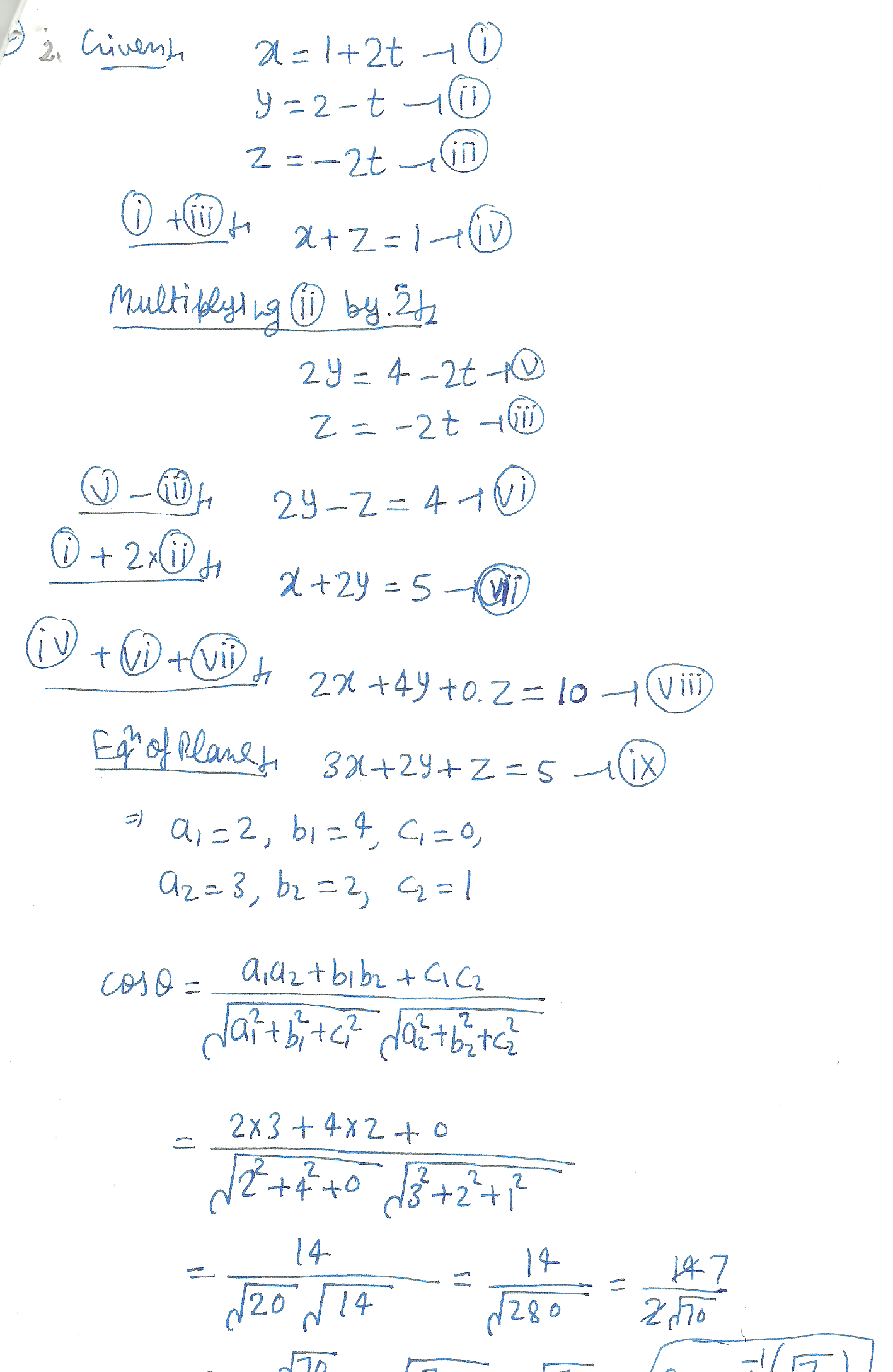4939

Mathematics Coordinate Geometry Level: Misc Level

What is the angle between the line L: x =1 +2t, y=2-t, z=-2t and the plane whose equation is 3x +2y+z =5